Subsections

# Maximal and Minimal Work

## Maximal Work

So far we discussed reversible processes. What happens for irreversible ones?

Let us discuss an insulated system (adiabatic process).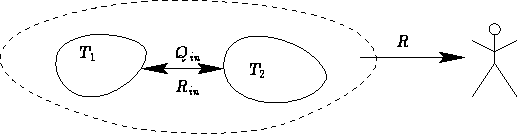We know the volume of the system in the final state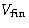. What is the maximal work R against the surroundings?

Since there is no heat exchange,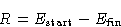(1)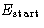is given. We want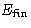to be as small as possible (we are greedy!). Since in final state the system is in equilibrium,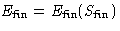. Differentiate (1):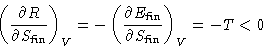R is a decreasing function of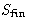. The lower is, the better! But S cannot decrease, it can only increase!

Conclusion:
R in a closed system is maximal for reversible processes, when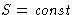Machines should produce as less entropy as possible!

## System and Environment

Consider a not insulated body at temperature T and pressure P surrounded by environment with temperature T0 and pressure P0: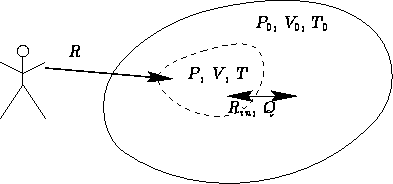What is the minimal work to change the state of the body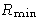?
• If the source makes work R, the body makes work -R
• Minimal work of the source corresponds to maximal work of the body

What is the change of energy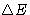of the body?

• Work from the external source R
• Work from the environment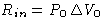• Heat from the environment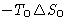We obtained: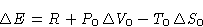• Since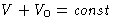,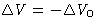• For reversible processes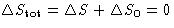, for irreversible processes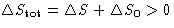. So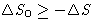Result: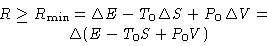(2)
Particular cases:
• T=T0,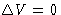(a non-insulated beaker):• T=T0, P=P0 (a non-insulated beaker under flexible stopper):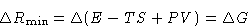• P=P0,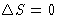(an insulated beaker under flexible stopper)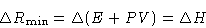Thermodynamic potentials describe the minimal work to produce the given state at certain external conditions.

## Thermodynamic Potentials and Irreversible Processes

Suppose now R=0. We obtained: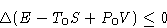This thing always decreases!
• If T=T0,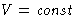, Helmholtz free energy A decreases,
• If T=T0, P=P0, Gibbs free energy G decreases,
• If P=P0,, enthalpy H decreases.

## Probability of a Fluctuation

Once again, a body in an environment (microcanonical ensemble):Let us consider it as a fluctuation: some macroscopic variable deviated from the mean value. Thenis the minimal work to create the fluctuation.

Probability for the fluctuation is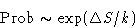In equilibrium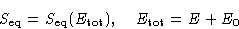When the system equilibrates, its total entropy increases, so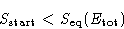Let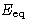be the energy for which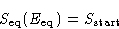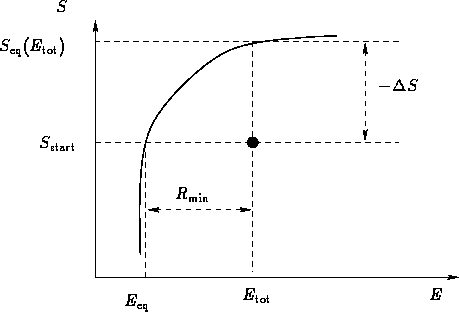Then: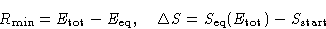Therefore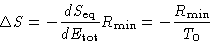We obtained: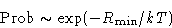(3)
Difference between (3) and Gibbs formulae: here we discuss the probability of a macroscopic state!

• Fluctuations at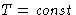,: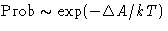• Fluctuations at,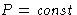: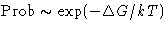• Fluctuations at,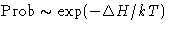Next: Thermodynamic Stability Up: Maximal Work, Minimal Work Previous: Maximal Work, Minimal Work

© 1997 Boris Veytsman and Michael Kotelyanskii
Fri Sep 12 00:09:21 EDT 1997